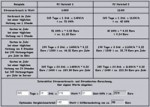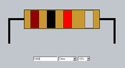# Interactive power consumption calculator – calculate current consumption and energy costsInteractive electricity costs calculator – calculate power consumption Calculate the current consumption and cost of electricity … With this simple online tool one can calculate fast and easy the current consumption and electricity costs. No matter, if one would like to calculate the computer power consumption or other household equipment. Simply change the values in the lower range and watch the approximate costs for your inputs. The interactive power consumption calculator and the examples are an excerpt from our last … Continue reading Interactive power consumption calculator – calculate current consumption and energy costs

# Interactive resistor to get the color codes for resistors within secondsIf you also don’t like boring color tables to find out the colors for your requested resistor, you can type in the desired resistor value, multiplier and tolerance to get them within seconds. Furthermore I’ve now also added a direct color choice, where it’s now possible to get the correct resistor value over the arrows of each color. This way you’re able to use the old resistors out of your drawer instead of buying new ones 😉 The new color … Continue reading Interactive resistor to get the color codes for resistors within seconds